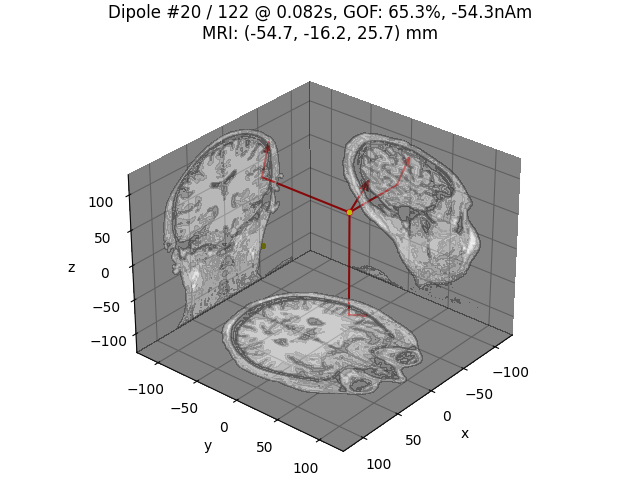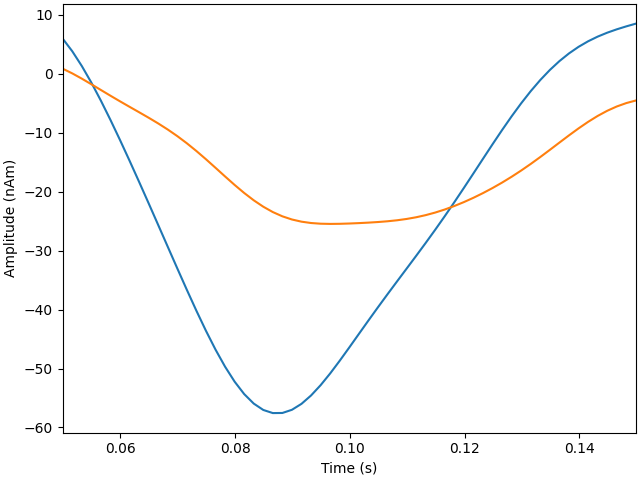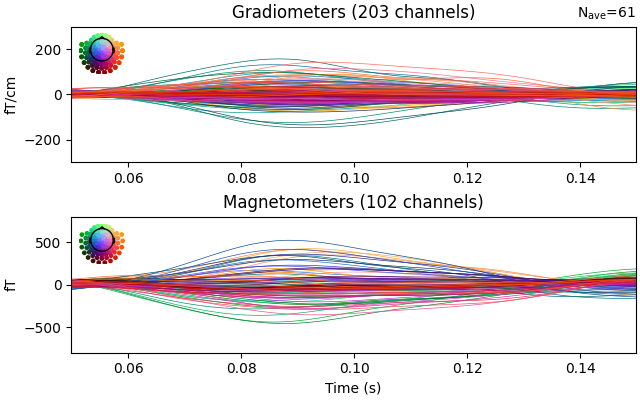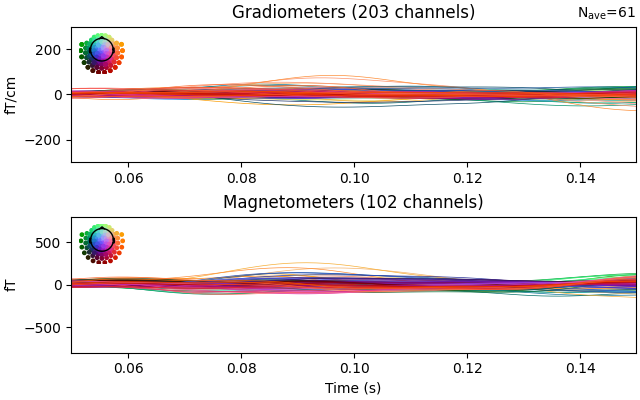# Compute Rap-Music on evoked data#

Compute a Recursively Applied and Projected MUltiple Signal Classification (RAP-MUSIC)  on evoked data.

```# Author: Yousra Bekhti <yousra.bekhti@gmail.com>
#
```
```import mne

from mne.datasets import sample
from mne.beamformer import rap_music
from mne.viz import plot_dipole_locations, plot_dipole_amplitudes

print(__doc__)

data_path = sample.data_path()
subjects_dir = data_path / 'subjects'
meg_path = data_path / 'MEG' / 'sample'
fwd_fname = meg_path / 'sample_audvis-meg-eeg-oct-6-fwd.fif'
evoked_fname = meg_path / 'sample_audvis-ave.fif'
cov_fname = meg_path / 'sample_audvis-cov.fif'

# Read the evoked response and crop it
condition = 'Right Auditory'
baseline=(None, 0))
# select N100
evoked.crop(tmin=0.05, tmax=0.15)

evoked.pick_types(meg=True, eeg=False)

dipoles, residual = rap_music(evoked, forward, noise_cov, n_dipoles=2,
return_residual=True, verbose=True)
plot_dipole_locations(dipoles, trans, 'sample', subjects_dir=subjects_dir)
plot_dipole_amplitudes(dipoles)

# Plot the evoked data and the residual.
evoked.plot(ylim=dict(grad=[-300, 300], mag=[-800, 800], eeg=[-6, 8]),
time_unit='s')
residual.plot(ylim=dict(grad=[-300, 300], mag=[-800, 800], eeg=[-6, 8]),
time_unit='s')
```
••••```Reading /home/circleci/mne_data/MNE-sample-data/MEG/sample/sample_audvis-ave.fif ...
Read a total of 4 projection items:
PCA-v1 (1 x 102) active
PCA-v2 (1 x 102) active
PCA-v3 (1 x 102) active
Average EEG reference (1 x 60) active
Found the data of interest:
t =    -199.80 ...     499.49 ms (Right Auditory)
0 CTF compensation matrices available
nave = 61 - aspect type = 100
Projections have already been applied. Setting proj attribute to True.
Applying baseline correction (mode: mean)
Removing projector <Projection | Average EEG reference, active : True, n_channels : 60>
Computing patch statistics...
[done]
Computing patch statistics...
[done]
Desired named matrix (kind = 3523) not available
Read MEG forward solution (7498 sources, 306 channels, free orientations)
Desired named matrix (kind = 3523) not available
Read EEG forward solution (7498 sources, 60 channels, free orientations)
Forward solutions combined: MEG, EEG
Source spaces transformed to the forward solution coordinate frame
366 x 366 full covariance (kind = 1) found.
Read a total of 4 projection items:
PCA-v1 (1 x 102) active
PCA-v2 (1 x 102) active
PCA-v3 (1 x 102) active
Average EEG reference (1 x 60) active
Computing inverse operator with 305 channels.
305 out of 366 channels remain after picking
Selected 305 channels
Whitening the forward solution.
Created an SSP operator (subspace dimension = 3)
Computing rank from covariance with rank=None
Using tolerance 3.3e-13 (2.2e-16 eps * 305 dim * 4.8  max singular value)
Estimated rank (mag + grad): 302
MEG: rank 302 computed from 305 data channels with 3 projectors
Setting small MEG eigenvalues to zero (without PCA)
Creating the source covariance matrix